# NHÀ khoa học nguyễn văN ĐẠo với lý thuyết dao đỘng và chuyểN ĐỘng hỗN ĐỘN

tải về 3.08 Mb.
 trang 6/11 Chuyển đổi dữ liệu 15.11.2017 Kích 3.08 Mb.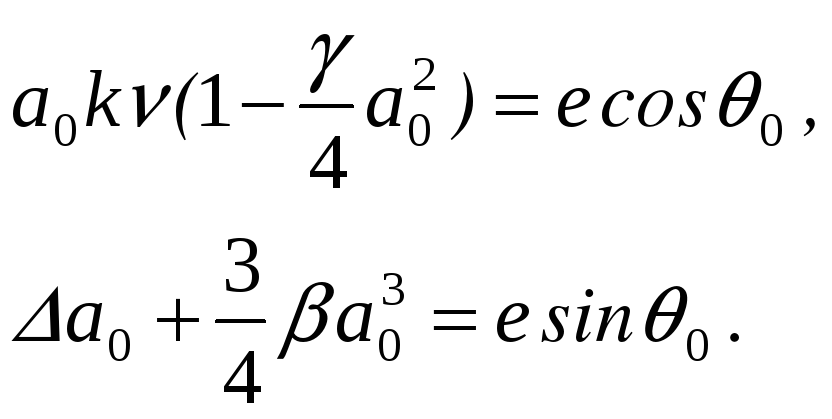(6) By eliminating the phase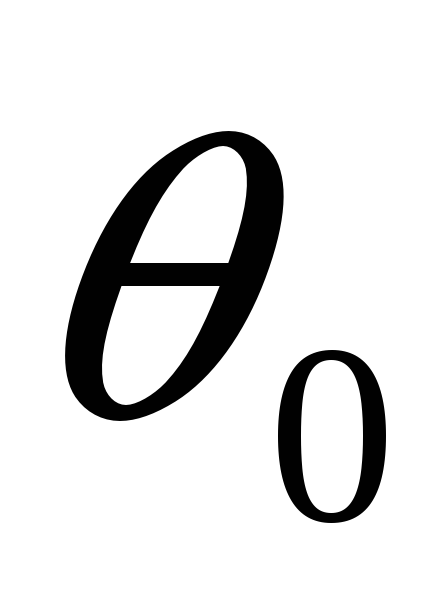from these equations we obtain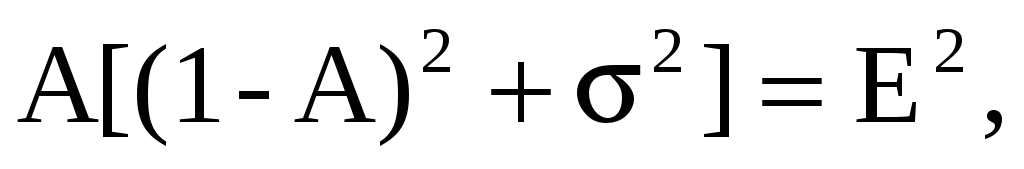(7) where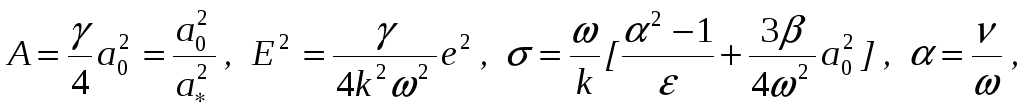(8)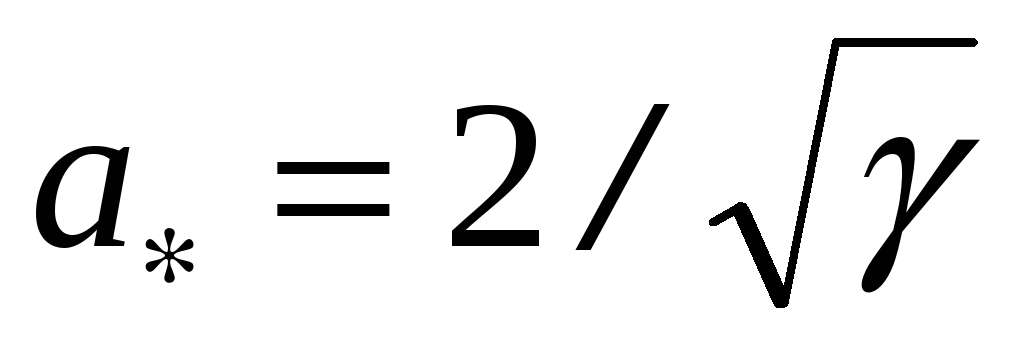is the amplitude of the purely self-excited van Der Pol oscillator. Below only the behaviour of forced oscillations with the frequencywhich is close towill be considered. The oscillation described by the equation (1) with stationary amplitude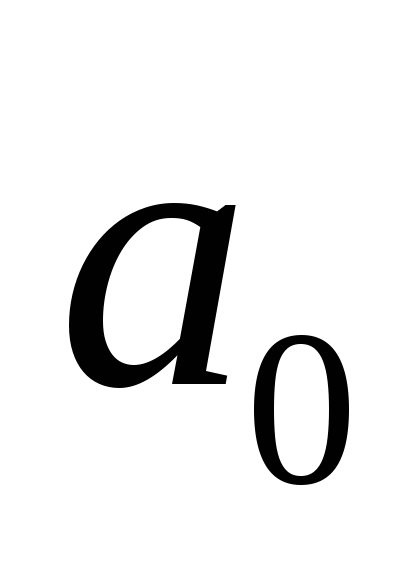and phase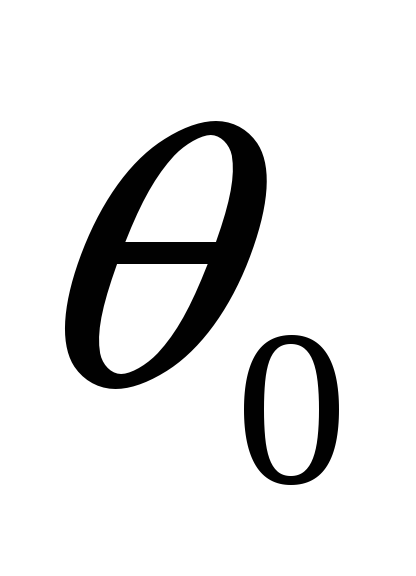: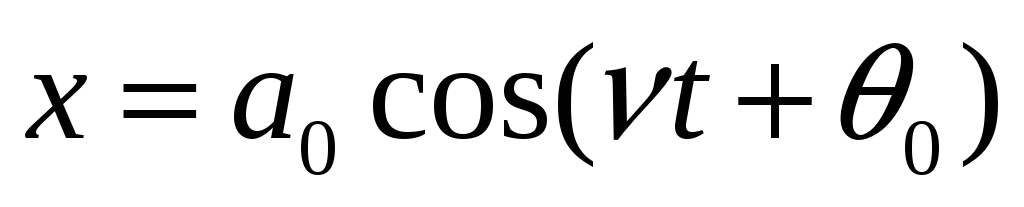has the frequency of the external forceonly. The self-excited oscillation is entrained by the external excitation .The synchronized oscillation (3) is characterized by the entrainment of the auto-periodic frequency by the external one.The synchronization effect is observed only when the exciting frequencyis close enough to the natural frequency. The amplitude curves with various values of external excitation (E) are given in the Figure 1 for the case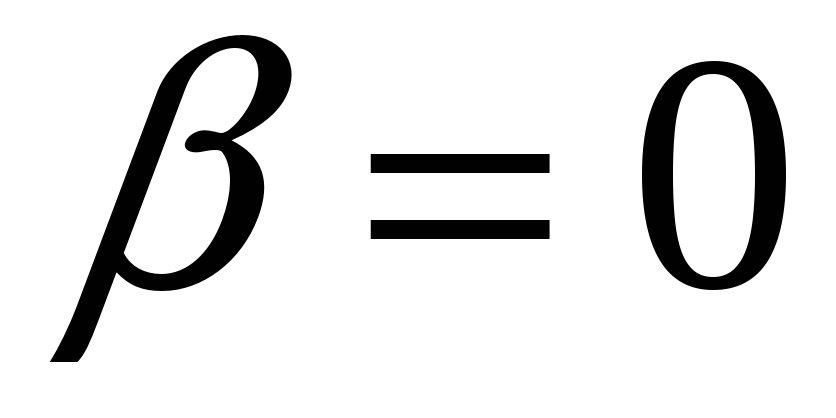. For E=0, i.e. for the zero external excitation, we find the results for the classical Van der Pol oscillator: 1) A=0 with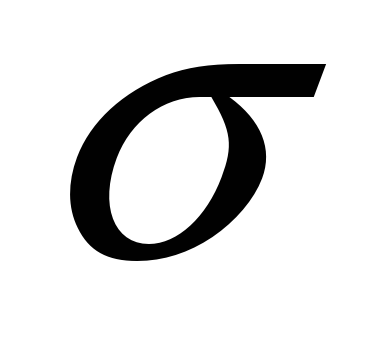arbitrary ,=0 , A=1. Therefore ,the resonance curves degenerate into the line A=0 (-axis) and the point=0,A=1 . If E is small but different from zero ,we expect A to be nearly 1 or nearly zero so that one of the response curves would be oval which is approximately the circle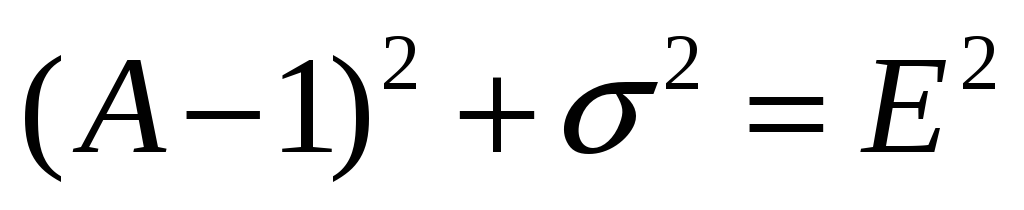with centre at=0 , A=1 . In addition, the other branch runs near the-axis .The oval expands with increasing E. When E increases, the resonance curves first consist of two branches, up to the critical value E=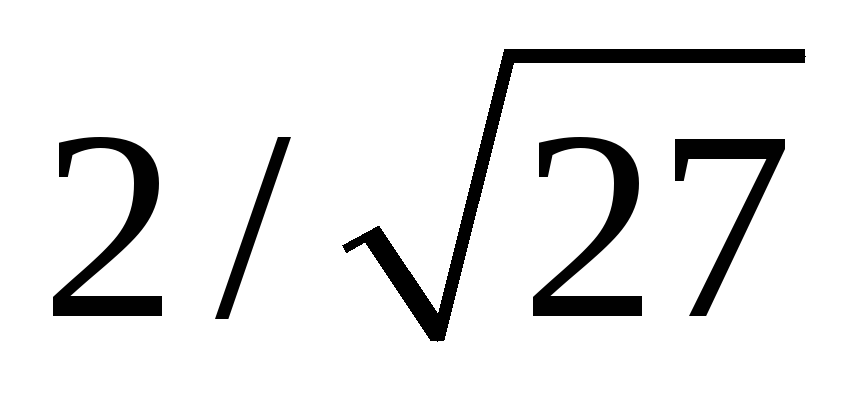for which the two branches join at=0, A=1/3, then with further increase of E the resonance curves have only a single branch . From the Figure 1 one can see that under certain conditions the frequency of the free oscillation is canceled out and is replaced by a synchronized oscillation, i.e. by an oscillation whose frequency is that of the external force ,namely: 1) For a given amplitude of the exciting force (E), the synchronization effect is observed when the exciting frequencyis close enough to the natural frequencyof the oscillator . The larger the amplitude of the exciting force ,the greater the frequency interval over which the synchronization occurs. For a given exciting frequency ,the oscillator is synchronized when the exciting amplitude is large enough . The closer the exciting frequency is to, the lower its threshold amplitude is.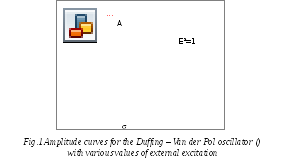Cơ sở dữ liệu được bảo vệ bởi bản quyền ©hocday.com 2019
được sử dụng cho việc quản lý

Quê hương